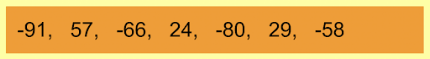Finding the Range 4
Set 4 (10 Questions)
Students collect numerical data and record, organize, display, and interpret the data on bar graphs and other representations:
Record numerical data in systematic ways, keeping track of what has been counted.
Identify features of data sets (range and mode).
This set largely focuses on the use of negative numbers in range calculation.
From Mr. Anker Tests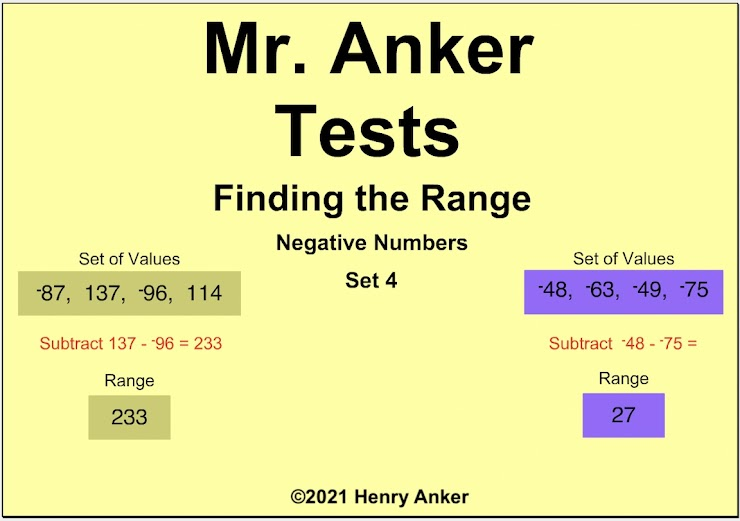Finding the Range Help Slide 1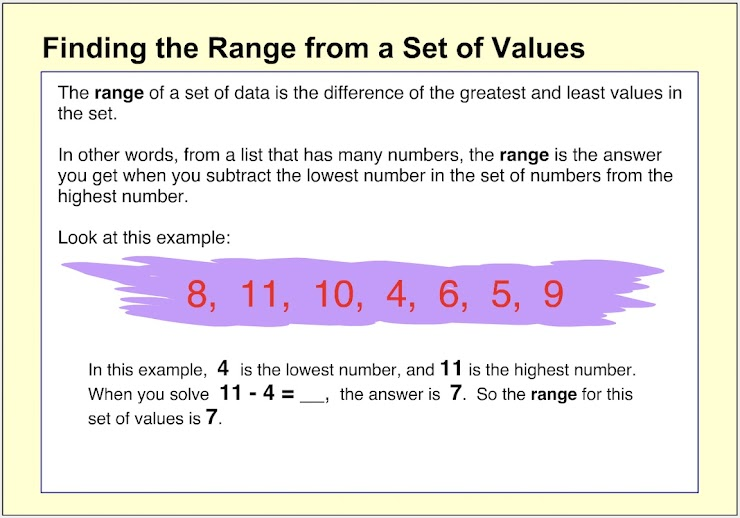Finding the Range Help Slide 2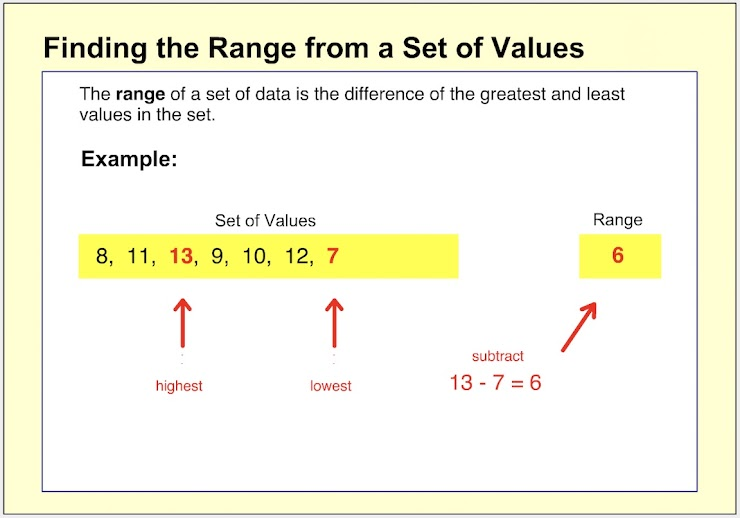Finding the Range Help Slide 3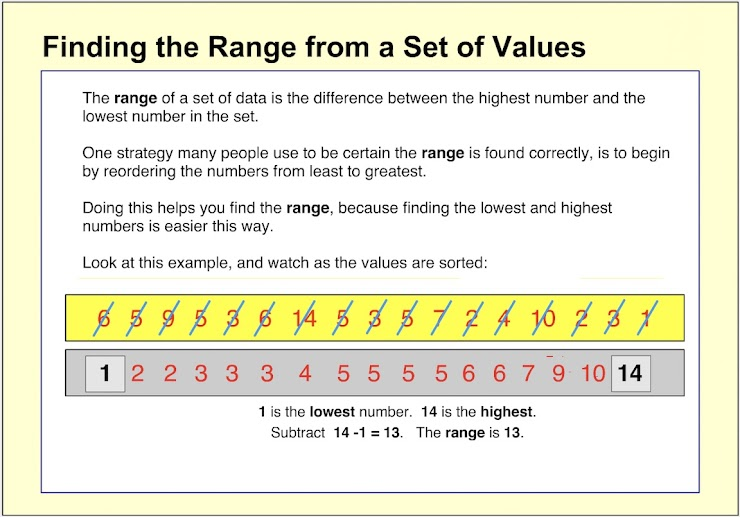Finding the Range Help Slide 4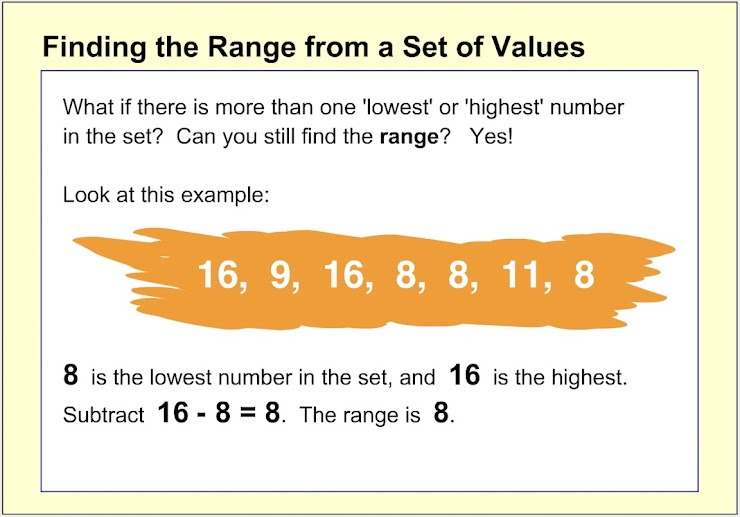Finding the Range Help Slide 5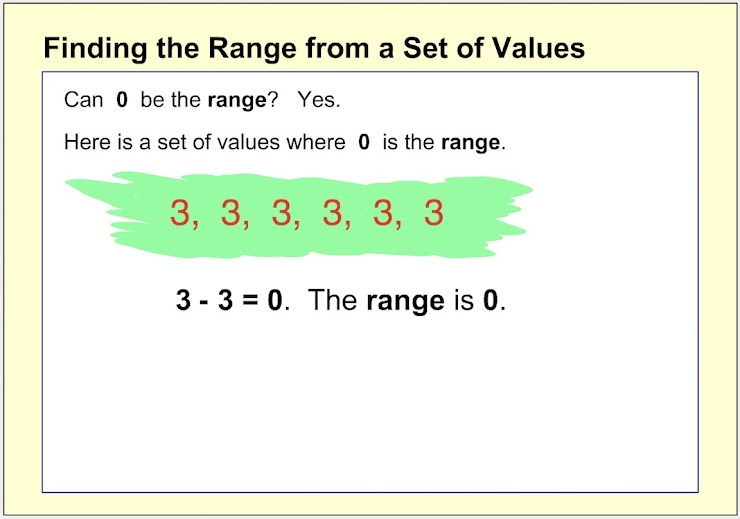Finding the Range Help Slide 6 (more advanced...)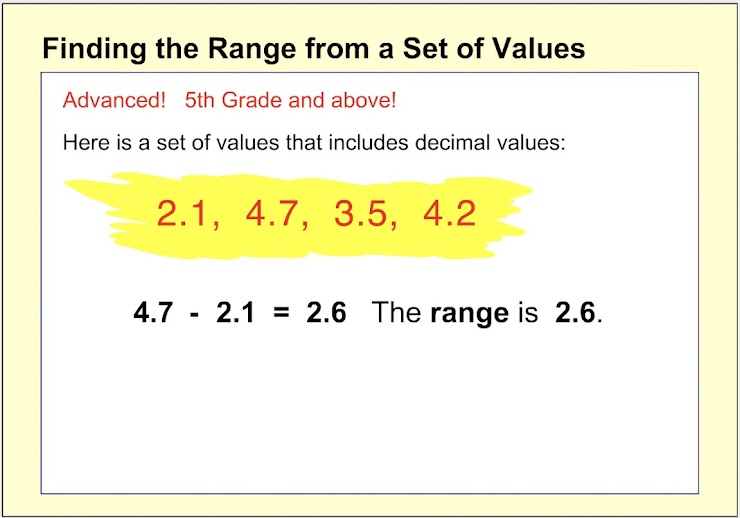Finding the Range Help Slide 7 (more advanced...)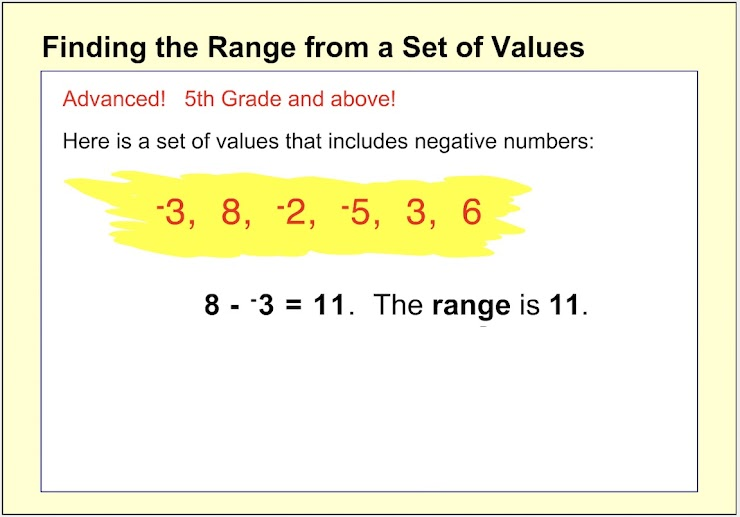1. Which choice below is the range for the set of values shown? *
1 point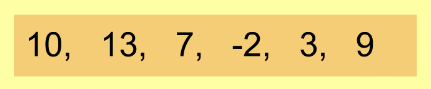Notice that the highest value is 13, and the lowest value in this set is a negative number, so the equation 13 - (-2) would be used to calculate the range.
2. Which choice below is the range for the set of values shown? *
1 point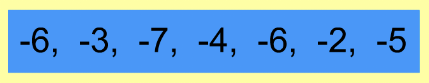Notice that the highest value is -2, and the lowest value in this set is -7, so the equation -2 - (-7) would be used to calculate the range.
3. Which choice below is the range for the set of values shown? *
1 point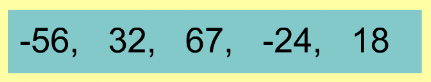4. Which choice below is the range for the set of values shown? *
1 point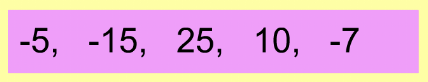5. On the line below, enter the range for the set of values shown. *
1 point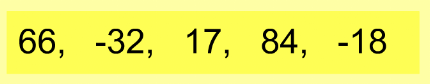6. On the line below, enter the range for the set of values shown. *
1 point7. Which of the choices below shows the range for the set of values shown? *
1 point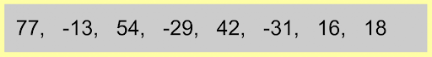8. Which choice below shows the correct equation to use to find the range? *
1 point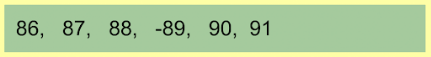9. On the line below, enter the range for the set of values shown. *
1 point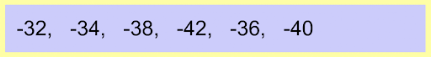10. On the line below, enter the range for the set of values shown. *
1 point